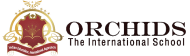Enquire Now

# Mass Calculator

Formula: Mass = Density x Volume
Leave the unknown field empty
What is Meant by Mass?
The quantity of matter within an object is called the mass of an object. The unit of measuring mass is often written in kilograms. The symbol that denotes mass is written as ‘m’.
What is the Mass calculation formula?
The formula for calculating mass is the multiplication of the density with volume.
Mass (m) = Density (ρ) x Volume (V)
How to Use the Mass Calculator?
The mass calculator gives accurate information on the topic for which you need to follow the steps given below:
Step 1: Type the values like the density and volume of the object.
Step 2: Click the ‘calculate’ button to get the object's mass.
The online mass calculator can help you to accurately calculate the mass of an object.
How to Calculate Mass?
Calculating mass with the density and volume of an object can be done using the formula given. Mass is calculated by multiplying the density and volume of the object.
Mass (m) = Density (ρ) x Volume (V)
Note: As per the international system of units (SI), the unit for density is measured in kilogram per cubic meter, or kg/m3, whereas volume is expressed in m3, and mass in kilogram (kg).
Example:The mass of iron is:
• Mass (m) = 55.84492768 kilogram
• Density (ρ) = 7874 cubic meter (m3 )
Steps:The mass of iron is:
• m = ρ × V
• = 7092.32 cubic centimeter × 7874 kilogram/cubic meter
• = 0.00709232 cubic meter × 7874 kilogram/cubic meter
• = 55.84492768 kilogram